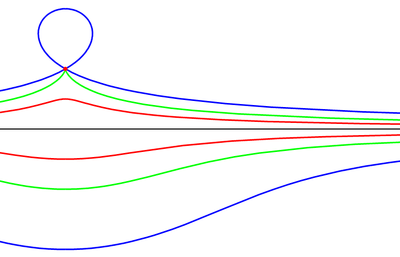# Conchoid (mathematics)Conchoids of line with common center.
The fixed point O is the red dot, the black line is the given curve, and each pair of coloured curves is length d from the intersection with the line that a ray through O makes. In the blue case d is greater than O's distance from the line, so the upper blue curve loops back on itself. In the green case d is the same, and in the red case it's less.

A conchoid is a curve derived from a fixed point O, another curve, and a length d.

## Description

For every line through O that intersects the given curve at A the two points on the line which are d from A are on the conchoid. The conchoid is, therefore, the cissoid of the given curve and a circle of radius d and center O. They are called conchoids because the shape of their outer branches resembles conch shells.

The simplest expression uses polar coordinates with O at the origin. Ifexpresses the given curve, thenexpresses the conchoid.

If the curve is a line, then the conchoid is the conchoid of Nicomedes.

For instance, if the curve is the line, then the line's polar form isand therefore the conchoid can be expressed parametrically asA limaçon is a conchoid with a circle as the given curve.

The so-called conchoid of de Sluze and conchoid of Dürer are not actually conchoids. The former is a strict cissoid and the latter a construction more general yet.Media related to Conchoid at Wikimedia Commons